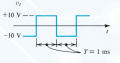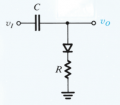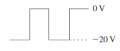# Determine Output Voltage Waveform for DC Restorer Circuit

#### ElectronicGuru

Joined Sep 26, 2014
22
I need to draw the output voltage waveform where the input voltage waveform is the following:The circuit is shown below:I recognize that this is a DC restorer circuit. When VI is high, the diode (assuming ideal diode model) will be a short, so we'll have a capacitor and resistor in series. When VI is low, the diode will be reverse biased, leaving us with an open circuit in which all of VI + VC will appear across Vo, assuming the capacitor is charged.

The solution for this problem is the following:I'm thrown off by this solution for two reason:

1. When the input waveform starts at -10 V, we have an open circuit. So all of the -10 V input should appear across this open circuit since we have not charged the capacitor yet, but the solution states that -20 V will appear across Vo initially. Perhaps I'm supposed to assume the capacitor is already charged, but if I don't, would I be correct in thinking that -10 V would be at the output initially?

2. When VI goes to 10 V, we'll have a short circuit through which the capacitor is charged. But then I'm thrown off by the resistor: when does it come into play with regard to exponential decay (RC circuit)? Does no decay occur here? If so, why?​

#### shteii01

Joined Feb 19, 2010
4,644
I see lots of thinking and guessing, and not enough math. Do the math.

#### crutschow

Joined Mar 14, 2008
27,935
........................
1. When the input waveform starts at -10 V, we have an open circuit. So all of the -10 V input should appear across this open circuit since we have not charged the capacitor yet, but the solution states that -20 V will appear across Vo initially. Perhaps I'm supposed to assume the capacitor is already charged, but if I don't, would I be correct in thinking that -10 V would be at the output initially?

2. When VI goes to 10 V, we'll have a short circuit through which the capacitor is charged. But then I'm thrown off by the resistor: when does it come into play with regard to exponential decay (RC circuit)? Does no decay occur here? If so, why?​
1. The solution is the steady-state solution after many cycles. It is not the initial waveform.

2. The resistor comes into play when the diode is forward bias. It determines the amount the capacitor charges each cycle until it reaches the steady-state value.

#### WBahn

Joined Mar 31, 2012
26,398
Assume that the frequency of the waveform is very low such that the period is many, many time constants. This will let you ignore the transients and focus on the steady state solution for each half of the input waveform.